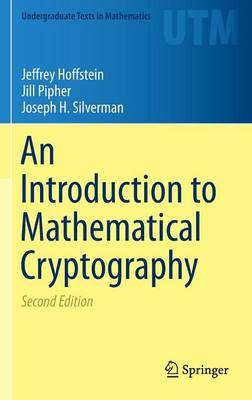•# An Introduction to Mathematical Cryptography - Undergraduate Texts in Mathematics (Hardback)

(author), (author), (author)
£62.99
Hardback 538 Pages
Published: 11/09/2014
• We can order this from the publisher

UK delivery within 3-4 weeks

This self-contained introduction to modern cryptography emphasizes the mathematics behind the theory of public key cryptosystems and digital signature schemes. The book focuses on these key topics while developing the mathematical tools needed for the construction and security analysis of diverse cryptosystems. Only basic linear algebra is required of the reader; techniques from algebra, number theory, and probability are introduced and developed as required. This text provides an ideal introduction for mathematics and computer science students to the mathematical foundations of modern cryptography. The book includes an extensive bibliography and index; supplementary materials are available online.

The book covers a variety of topics that are considered central to mathematical cryptography. Key topics include:

classical cryptographic constructions, such as Diffie-Hellmann key exchange, discrete logarithm-based cryptosystems, the RSA cryptosystem, and digital signatures;

fundamental mathematical tools for cryptography, including primality testing, factorization algorithms, probability theory, information theory, and collision algorithms;

an in-depth treatment of important cryptographic innovations, such as elliptic curves, elliptic curve and pairing-based cryptography, lattices, lattice-based cryptography, and the NTRU cryptosystem.

The second edition of An Introduction

to Mathematical Cryptography includes a significant revision of the material on digital signatures, including an earlier introduction to RSA, Elgamal, and DSA signatures, and new material on lattice-based signatures and rejection sampling. Many sections have been rewritten or expanded for clarity, especially in the chapters on information theory, elliptic curves, and lattices, and the chapter of additional topics has been expanded to include sections on digital cash and homomorphic encryption. Numerous new exercises have been included.

Publisher: Springer-Verlag New York Inc.
ISBN: 9781493917105
Number of pages: 538
Weight: 9516 g
Dimensions: 235 x 155 x 30 mm
Edition: 2nd ed. 2014

MEDIA REVIEWS

"This book explains the mathematical foundations of public key cryptography in a mathematically correct and thorough way without omitting important practicalities. ... I would like to emphasize that the book is very well written and quite clear. Topics are well motivated, and there are a good number of examples and nicely chosen exercises. To me, this book is still the first-choice introduction to public-key cryptography." (Klaus Galensa, Computing Reviews, March, 2015)

"This is a text for an upper undergraduate/lower graduate course in mathematical cryptography. ... It is very well written and quite clear. Topics are well-motivated, and there are a good number of examples and nicely chosen exercises. ... An instructor of a fairly sophisticated undergraduate course in cryptography who wants to emphasize public key cryptography should definitely take a look at this book." (Mark Hunacek, MAA Reviews, October, 2014)